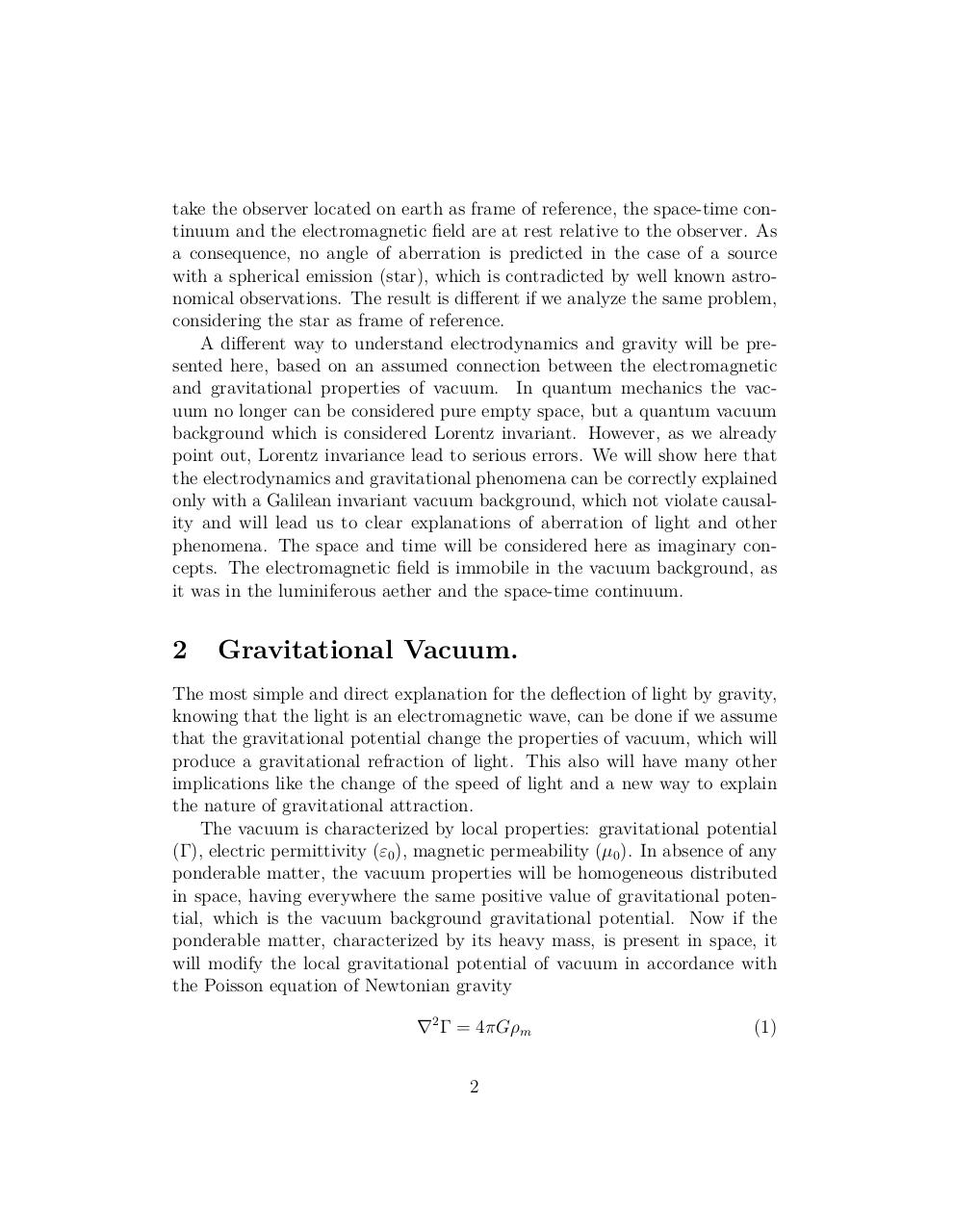# gvac art.pdfPage 1 23425

#### Text preview

take the observer located on earth as frame of reference, the space-time continuum and the electromagnetic field are at rest relative to the observer. As
a consequence, no angle of aberration is predicted in the case of a source
with a spherical emission (star), which is contradicted by well known astronomical observations. The result is different if we analyze the same problem,
considering the star as frame of reference.
A different way to understand electrodynamics and gravity will be presented here, based on an assumed connection between the electromagnetic
and gravitational properties of vacuum. In quantum mechanics the vacuum no longer can be considered pure empty space, but a quantum vacuum
background which is considered Lorentz invariant. However, as we already
point out, Lorentz invariance lead to serious errors. We will show here that
the electrodynamics and gravitational phenomena can be correctly explained
only with a Galilean invariant vacuum background, which not violate causality and will lead us to clear explanations of aberration of light and other
phenomena. The space and time will be considered here as imaginary concepts. The electromagnetic field is immobile in the vacuum background, as
it was in the luminiferous aether and the space-time continuum.

2

Gravitational Vacuum.

The most simple and direct explanation for the deflection of light by gravity,
knowing that the light is an electromagnetic wave, can be done if we assume
that the gravitational potential change the properties of vacuum, which will
produce a gravitational refraction of light. This also will have many other
implications like the change of the speed of light and a new way to explain
the nature of gravitational attraction.
The vacuum is characterized by local properties: gravitational potential
(Γ), electric permittivity (ε0 ), magnetic permeability (µ0 ). In absence of any
ponderable matter, the vacuum properties will be homogeneous distributed
in space, having everywhere the same positive value of gravitational potential, which is the vacuum background gravitational potential. Now if the
ponderable matter, characterized by its heavy mass, is present in space, it
will modify the local gravitational potential of vacuum in accordance with
the Poisson equation of Newtonian gravity
∇2 Γ = 4πGρm
2

(1)